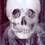# How Fast Can You Multiply?

Multiply: 14 $$\times$$ 12 $$\times$$ 1,000 $$\times$$ $$\frac{4}{7}$$ $$\times$$ $$\frac{13}{1}$$ $$\times$$ 0 $$\times$$ $$\frac{5}{6}$$ $$\times$$ 32 $$\times$$ 1 $$\times$$ 4 $$\times$$ $$\sqrt{5}$$ $$\times$$ 2 $$\times$$ $$\frac{3}{4}$$ $$\times$$ 2$$\sqrt{5}$$ $$\times$$ 9 $$\times$$ 32Note by Kaleil Salomon -Jacob
7 years, 5 months ago

This discussion board is a place to discuss our Daily Challenges and the math and science related to those challenges. Explanations are more than just a solution — they should explain the steps and thinking strategies that you used to obtain the solution. Comments should further the discussion of math and science.

When posting on Brilliant:

• Use the emojis to react to an explanation, whether you're congratulating a job well done , or just really confused .
• Ask specific questions about the challenge or the steps in somebody's explanation. Well-posed questions can add a lot to the discussion, but posting "I don't understand!" doesn't help anyone.
• Try to contribute something new to the discussion, whether it is an extension, generalization or other idea related to the challenge.

MarkdownAppears as
*italics* or _italics_ italics
**bold** or __bold__ bold
- bulleted- list
• bulleted
• list
1. numbered2. list
1. numbered
2. list
Note: you must add a full line of space before and after lists for them to show up correctly
paragraph 1paragraph 2

paragraph 1

paragraph 2

[example link](https://brilliant.org)example link
> This is a quote
This is a quote
    # I indented these lines
# 4 spaces, and now they show
# up as a code block.

print "hello world"
# I indented these lines
# 4 spaces, and now they show
# up as a code block.

print "hello world"
MathAppears as
Remember to wrap math in $$ ... $$ or $ ... $ to ensure proper formatting.
2 \times 3 $2 \times 3$
2^{34} $2^{34}$
a_{i-1} $a_{i-1}$
\frac{2}{3} $\frac{2}{3}$
\sqrt{2} $\sqrt{2}$
\sum_{i=1}^3 $\sum_{i=1}^3$
\sin \theta $\sin \theta$
\boxed{123} $\boxed{123}$

Sort by:

Well it's 0, obviously

- 7 years, 5 months ago

Yeah, whenever I see things like this I always look for two things, reciprocals and zeros. Then the whole thing is a trick. :)

- 7 years, 5 months ago

in 0.000000000000000000000000000000000001 Sec its 0

- 7 years, 5 months ago

0

- 7 years, 5 months ago

0

- 7 years, 5 months ago

It is of course 0. ( I have faced this trick many times!)

- 7 years, 5 months ago

first of all why r u putting a trick here and its 000000000000000000000 duh

- 7 years ago

0

- 7 years, 5 months ago